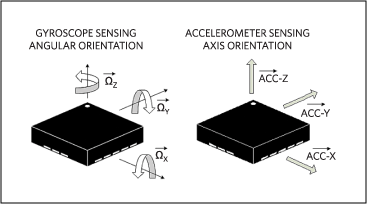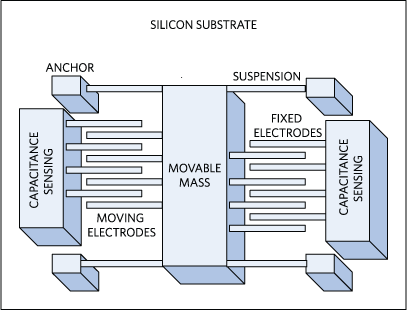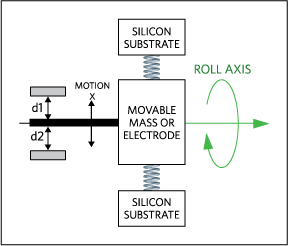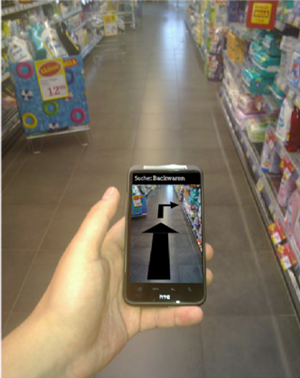# Accelerometer and Gyroscopes Sensors: Operation, Sensing, and Applications

### Abstract

Microelectromechanical systems (MEMS) in consumer electronics are growing faster each year, with increasing demands from the mobile market, which is dominating the growth for this emerging technology. MEMS sensors are, in fact, becoming the key elements in designing differentiating products for consumer and mobile markets like game consoles, smartphones, and tablets. MEMS give the user a new way to interface with their smart device. This paper is an overview of MEMS: the principle of their operation, the sensing mechanism, and a variety of potential applications.

### Introduction

Microelectromechanical systems (MEMS) combine mechanical and electrical components into small structures in the micrometer scale. They are formed by a combination of semiconductor and microfabrication technologies using micro machine processing to integrate all the electronics, sensors, and mechanical elements onto a common silicon substrate. Major components in any MEMS system are the mechanical elements, sensing mechanism, and the ASIC or a microcontroller. This article presents an overview of MEMS accelerometer sensors and gyroscopes. We discuss the principles of their operation, their sensing mechanism, the growing variety of applications for them, and the profound impact they are already having on our daily lives.

### MEMS as Inertial Sensors

MEMS sensors have many applications in measuring either linear acceleration along one or several axis, or angular motion about one or several axis as an input to control a system (Figure 1).All MEMS accelerometer sensors commonly measure the displacement of a mass with a position-measuring interface circuit. That measurement is then converted into a digital electrical signal through an analog-to-digital converter (ADC) for digital processing. Gyroscopes, however, measure both the displacement of the resonating mass and its frame because of the Coriolis acceleration.

### Basic Accelerometer Operation

Newton’s Second law of motion says that the acceleration (m/s2) of a body is directly proportional to, and in the same direction as, the net force (Newton) acting on the body, and inversely proportional to its mass (gram).

Acceleration = Force (Newton)
(m/s2) Mass (gram)

It is important to note that acceleration creates a force that is captured by the force-detection mechanism of the accelerometer. So the accelerometer really measures force, not acceleration; it basically measures acceleration indirectly through a force applied to one of the accelerometer's axes.

An accelerometer is also an electromechanical device, including holes, cavities, springs, and channels, that is machined using microfabrication technology. Accelerometers are fabricated in a multilayer wafer process, measuring acceleration forces by detecting the displacement of the mass relative to fixed electrodes.

### The Accelerometer's Sensing Mechanism

A common sensing approach used in accelerometers is capacitance sensing in which acceleration is related to change in the capacitance of a moving mass (Figure 2). This sensing technique is known for its high accuracy, stability, low power dissipation, and simple structure to build. It is not prone to noise and variation with temperature. Bandwidth for a capacitive accelerometer is only a few hundred Hertz because of their physical geometry (spring) and the air trapped inside the IC that acts as a damper.

C = (ε0 × εr × A)/D (Farad)

ε0 = Permitted free space
εr = Relative material permitted between plates
A = Area of overlap between electrodes
D = Separation between the electrodesThe capacitance can either be arranged as single-sided or a differential pair. Let’s look at accelerometers arranged as a differential pair (Figure 3). It is composed of a single movable mass (one planar surface), that is placed along with a mechanical spring between two, fixed, reference silicon substrates or electrodes (another planar surface). It is obvious that the movement of the mass (Motion x) is relative to the fixed electrodes (d1 and d2), and causes a change in capacitances (C1 and C2). By calculating the difference between C2 and C1 we can derive the displacement of our mass and its direction.The displacement of the movable mass (micrometer) is caused by acceleration, and it creates an extremely small change in capacitance for proper detection (Equation 1). This mandates using multiple movable and fixed electrodes, all connected in a parallel configuration. The configuration enables a greater change in capacitance, which can both be detected more accurately, and ultimately makes capacitance sensing a more feasible technique.

Let’s summarize quickly. Force causes a displacement of the mass which, in return, causes a capacitance change. Now, placing multiple electrodes in parallel allows a larger capacitance, which will be more easily detected (Figure 4). V1 and V2 are electrical connections to each side of the capacitors and form a voltage-divider with the center point as the voltage of our mass.The analog mass voltage will go through charge amplification, signal conditioning, demodulation, and lowpass filtering before it gets converted into a digital domain using a sigma-delta ADC. The serial digital bit stream from the ADC is then passed to a FIFO buffer that converts the serial signal into a parallel data stream. That parallel data stream can then be transformed using a serial protocol like I2C or SPI before it is sent to the host for further processing (Figure 5).A sigma-delta ADC is well suited for accelerometer applications because of its low signal bandwidth and high resolution. With an output value defined by its number of bits, a sigma-delta ADC can be translated into “g” units for an accelerometer application very easily. The “g” is a unit of acceleration equal to the earth’s gravity at sea level:

For example, if the X-axis reading of our 10-bit ADC is equal to 600 out of the available 1023 (210 - 1 = 1023), and with 3.3V as the reference, we can derive the voltage for the X-axis specified in “g“ with the following equation:

X - voltage = (600 × 3.3)/1023 = 1.94V

Each accelerometer has a zero-g voltage level that is the voltage that corresponds to 0g. We first calculate the voltage shifts from zero-g voltage (specified in the data sheet and assumed to be 1.65V) as:

1.94V - 1.65V = 0.29V

Now, to do the final conversion we divide 0.29V by the accelerometer’s sensitivity (specified in the data sheet and assumed to be 0.475V/g):

0.29V/0.475V/g = 0.6g

### A Multiaxis Accelerometer

Let’s take another look at our Figure 3 and add an actual manufactured accelerometer (Figure 6). Now we can clearly relate each component of an accelerometer to its mechanical model.By simply mounting an accelerometer differently (90 degrees, shown in Figure 7) we can create a 2-axis accelerometer needed for more sophisticated applications.There are two ways to construct a two-axis accelerometer: lay out the two different single-axis accelerometer sensors perpendicular to each other, or use a single mass with capacitive sensors arranged to measure movement along both axes.

### Selecting an Accelerometer

When selecting an accelerometer for a given application, it is important to consider some of its key characteristics:

1. Bandwidth (Hz): the bandwidth of a sensor indicates the range of vibration frequencies to which the accelerometer responds or how often a reliable reading can be taken. Humans cannot create body motion much beyond the range of 10Hz to12Hz. For this reason, a bandwidth of 40Hz to 60Hz is adequate for sensing a tilt or human motion.
2. Sensitivity (mV/g or LSB/g): sensitivity is a measure of the minimum detectable signal or the change in output electrical signal per change in input mechanical change. This is valid in one frequency only.
3. Voltage noise density (µg/SQRT Hz): voltage noise changes with the inverse square root of the bandwidth. The faster that we read accelerometer changes, the worse accuracy we get. Noise has a higher influence on the performance of the accelerometers when operating at lower g conditions with a smaller output signal.
4. Zero-g voltage: this term specifies the range of voltages that can be expected at the output under 0g of acceleration.
5. Frequency response (Hz): this is the frequency range specified with a tolerance band (±5%, etc) for which the sensor will detect motion and report a true output. The specified band tolerance lets the user calculate how much the device's sensitivity deviates from the reference sensitivity at any frequency within its specified frequency range.
6. Dynamic range (g): this is the range between the smallest detectable amplitude that the accelerometer can measure to the largest amplitude before distorting or clipping the output signal.

### Accelerometer Versus Gyroscope

Before describing some MEMS applications, we must understand the differences between an accelerometer and a gyroscope. Accelerometers measure linear acceleration (specified in mV/g) along one or several axis. A gyroscope measures angular velocity (specified in mV/deg/s). If we take our accelerometer and impose a rotation to it (i.e., a roll) (Figure 8), the distances d1 and d2 will not change. Consequently the accelerometer’s output will not respond to change in angular velocity.We can construct the sensor differently, so the inner frame containing the resonating mass is connected to the substrate by springs at 90 degrees relative to the resonating motion (Figure 9). Then we can measure Coriolis acceleration though capacitance sensing on the electrodes mounted between the inner frame and substrate.### Accelerometer and Gyroscope Applications

Accelerometers have been used for a long time in automobiles for detecting car crashes and for triggering airbags at just the right moment. They have many applications in mobile devices like switching between portrait and landscape modes, tap gestures to change to the next song, tapping through clothing when the device is in a pocket, or anti-blur capturing and optical image stabilization.

Acceleration is the rate of change of velocity

α = δv/δ t = δ2x/δt2

We can derive velocity and distance information from the acceleration output by single or double integration, respectively. By adding the measurements provided by gyroscopes, we can then use a special technique to track the position and orientation of an object relative to a known starting point. This information is used for indoor navigation without external reference or GPS signal (Figure 10).#### Optical Image Stabilization

Human hands shake at a very low frequency (10Hz to 20Hz). When taking a picture with our latest small, lightweight smartphones and cameras, we cause jitter that blurs the image. Features like optical zoom aggravate this problem and produce even more blurring.

Consider a sensor with 0.08 degree horizontal drift when using an SVGA camera with 800x600 pixel resolution for a 45 degree viewing angle. The 45/800 = 0.056 degree, which corresponds to 1.42 pixel blurring. As the camera resolution improves, the blurring covers more pixels and causes more image distortion.Gyroscope-based optical image stabilization (Figure 11) with correctional software can compensate for image blurring by sending a mechanical gyroscope’s measured data to a microcontroller and linear motor to move the image sensor.

#### Gesture-Based Control

We can use MEMS accelerometer sensors for gesture-based control of a wireless mouse, or wheel chair directional control, or a gyroscope in a Wii® console. Other examples include a smart device using gestures to control a cursor on TV, or “virtual” knobs, or even gesture commands to control external devices with a handheld wireless sensor unit.

### Conclusion

MEMS accelerometer sensors and gyroscopes have long been used for a wide range of applications in shipping, space, industrial robotics, and automobiles. But their application versatility has now spread to smartphones where they give us a new way to interface for motion and gestures with our smart device. Understanding MEMS behavior and the characteristics of an accelerometer or gyroscope allows designers to design more efficient and low-cost products for high-volume applications. These MEMS devices also allow us to create new applications that are profoundly changing how our movements, body motion, and gestures impact the way we live.